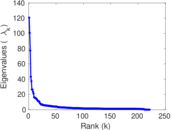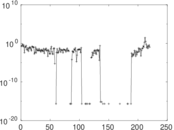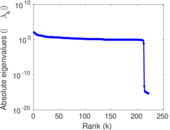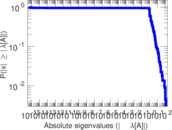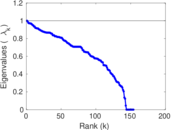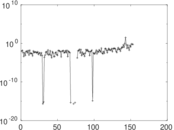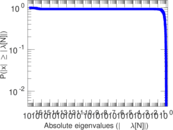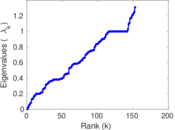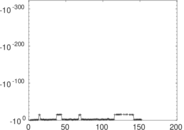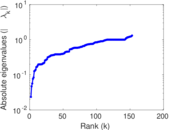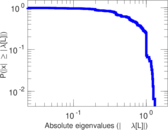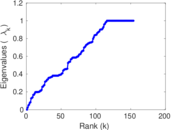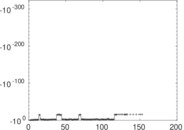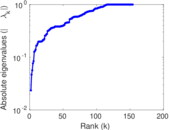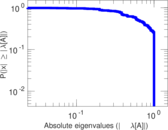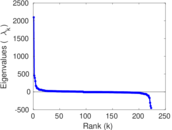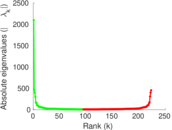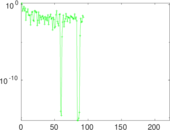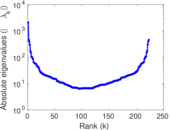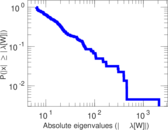# Wikibooks edits (ms)

This is the bipartite edit network of the Malay Wikibooks. It contains users and pages from the Malay Wikibooks, connected by edit events. Each edge represents an edit. The dataset includes the timestamp of each edit.

 Code `bms` Internal name `edit-mswikibooks` Name Wikibooks edits (ms) Data source http://dumps.wikimedia.org/ AvailabilityDataset is available for download Consistency checkDataset passed all tests Category Authorship network Dataset timestamp 2017-10-20 Node meaning User, article Edge meaning Edit Network formatBipartite, undirected Edge typeUnweighted, multiple edges Temporal dataEdges are annotated with timestamps

## Statistics

 Size n = 2,102 Left size n1 = 223 Right size n2 = 1,879 Volume m = 5,593 Unique edge count m̿ = 2,879 Wedge count s = 566,847 Claw count z = 151,699,990 Cross count x = 34,482,133,099 Square count q = 37,610 4-Tour count T4 = 2,574,142 Maximum degree dmax = 1,744 Maximum left degree d1max = 1,744 Maximum right degree d2max = 210 Average degree d = 5.321 60 Average left degree d1 = 25.080 7 Average right degree d2 = 2.976 58 Fill p = 0.006 870 84 Average edge multiplicity m̃ = 1.942 69 Size of LCC N = 1,890 Diameter δ = 11 50-Percentile effective diameter δ0.5 = 3.212 36 90-Percentile effective diameter δ0.9 = 5.132 96 Median distance δM = 4 Mean distance δm = 3.538 50 Gini coefficient G = 0.729 691 Balanced inequality ratio P = 0.217 325 Left balanced inequality ratio P1 = 0.114 429 Right balanced inequality ratio P2 = 0.302 879 Relative edge distribution entropy Her = 0.779 297 Power law exponent γ = 3.604 70 Tail power law exponent γt = 2.951 00 Tail power law exponent with p γ3 = 2.951 00 p-value p = 0.000 00 Left tail power law exponent with p γ3,1 = 1.731 00 Left p-value p1 = 0.462 000 Right tail power law exponent with p γ3,2 = 5.651 00 Right p-value p2 = 0.001 000 00 Degree assortativity ρ = −0.130 732 Degree assortativity p-value pρ = 1.897 73 × 10−12 Spectral norm α = 120.737 Algebraic connectivity a = 0.023 444 2 Spectral separation |λ1[A] / λ2[A]| = 1.199 22 Controllability C = 1,649 Relative controllability Cr = 0.794 699

## Plots

### Fruchterman–Reingold graph drawing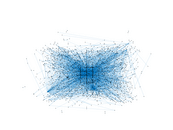### Degree distribution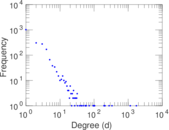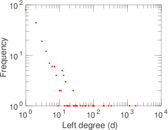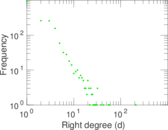### Cumulative degree distribution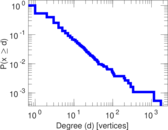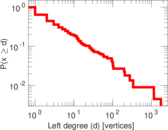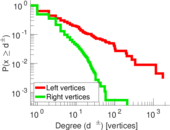### Lorenz curve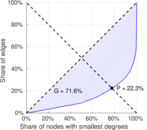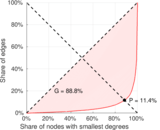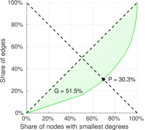### Spectral distribution of the adjacency matrix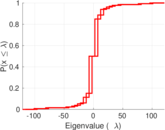### Spectral distribution of the normalized adjacency matrix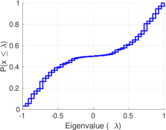### Spectral distribution of the Laplacian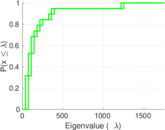### Spectral graph drawing based on the adjacency matrix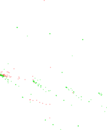### Spectral graph drawing based on the Laplacian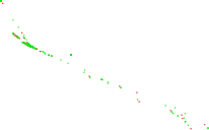### Spectral graph drawing based on the normalized adjacency matrix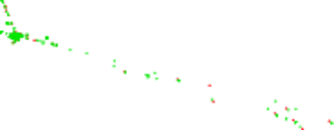### Degree assortativity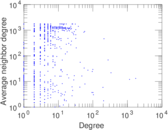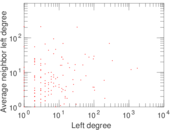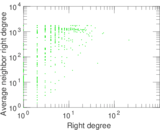### Zipf plot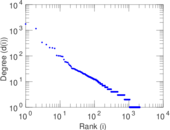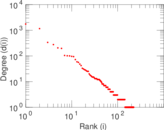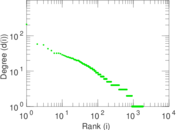### Hop distribution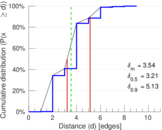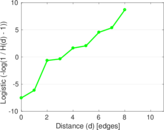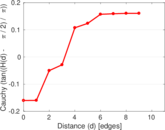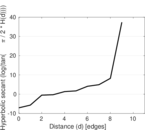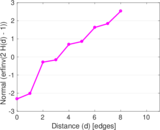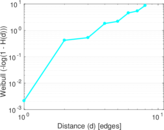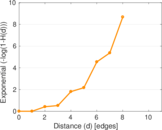### Double Laplacian graph drawing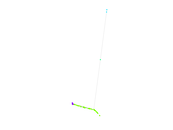### Delaunay graph drawing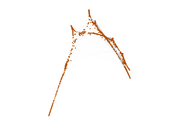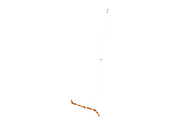### Edge weight/multiplicity distribution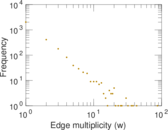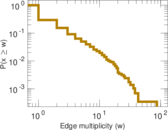### Temporal distribution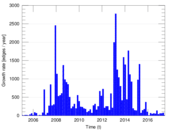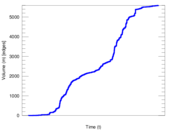### Temporal hop distribution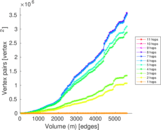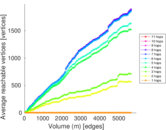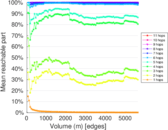### Diameter/density evolution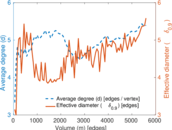### Matrix decompositions plots